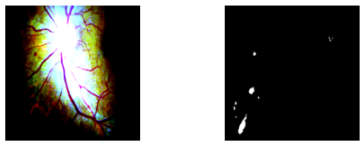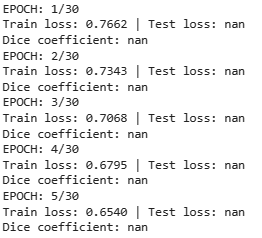# Normalize image pretrained resnet34 encoder U-net

i use unet with pretrained resnet34 as encoder to segment diabetic retinopathy lession, before training, do i have to normalize the image with mean=[0.485, 0.456, 0.406] and std=[0.229, 0.224, 0.225] as its documentation? Because the result image get darken on the edge and i’m sure it’s affecting in generating the predicted mask, thank you for helping!

The `Normalize` transformation is used to create a zero-mean and unit-variance input to the model.
The specified `mean` and `std` values were calculated from the `ImageNet` dataset and are often applied to images coming from a similar data distribution. However, since you are working with medical data I would assume the `mean` and `std` might differ and you might thus need to calculate these stats from your training dataset.

ah thankyou! since i’m newbie in pytorch, how can i calculate those value from my dataset?

You could either directly calculate the `mean` and `std` in case you are preloading the data or you could iterate the `DataLoader` and calculate these stats from each batch as seen in e.g. this post.

thankyou, i’ve tried to calculate mean and std and get the result, then i applied it on my dataset. after that, i checked one of my image dataset still get darken on the edge like this(left:fundus image, right:groundtruth)
Is there any other way to normalize dataset beside using mean and std?

I don’t know what the issue is since normalizing the image might distort its visualization.
Depending on the window you are using you might clip the image values so could you describe your concerns a bit more, please?

hm, i’m confused too, but here’s my code to show some of my dataset

``````import matplotlib.pyplot as plt

dataset = ProcessDataset(train_x, train_y)

fig, axs = plt.subplots(nrows=5, ncols=2, figsize=(8, 16))
for i, (data, target) in enumerate(dataset):
if i >= 5:
break
axs[i, 0].imshow(data.permute(1, 2, 0), cmap='gray')
axs[i, 1].imshow(np.squeeze(target), cmap='gray')
axs[i, 0].axis('off')
axs[i, 1].axis('off')
plt.show()
``````

and the output is like my previous answer, and talk about clip value, i got a warning

WARNING:matplotlib.image:Clipping input data to the valid range for imshow with RGB data ([0…1] for floats or [0…255] for integers).

The warning indicates that the normalized image was clipped, which could distort the visualization. Do you see any other issue using these normalized images during training of your model?

yes, test loss and dice coefficient giving nan resultThis seems to be related to this topic and thus I assume unrelated to the normalization?

oh so the normalization before training isn’t related with test loss and dice coefficient?